## (Solved) : Checkinventory Application Java Part 1 Implement Checkinventory Application Port Hueneme Q37222888 . . .

• I. CheckInventory Application in java

Part 1 – Implement CheckInventoryApplication

Port Hueneme receives a shipment of cars. Each car has adistinct id that consists of 3 letters in upper case (representedby a String), followed by a space, followed by 14 digits(represented by a long). As the cars are being unloaded from theship their IDs are recorded and placed in sorted order in a file.The file is sent to the dealer. The cars are driven to thedealership by designated drivers and arrive at the destination inrandom order. The car IDs are recorded at the dealership. Your taskis to compare the files and detect if they contain the sameelements utilizing hashing technique with O(n) complexity.

For example, the following two arrays have the same set ofelements (notice that duplicates are allowed):

A = {2,5,6,8,10,2,2}

B = {2,5,5,8,10,5,6}

To check if they have the same elements with hash table,consider the following algorithm:

1. Construct the hash table with array A elementsas keys:
• while inserting the elements, keep track of frequency for eachnumber in the key’s value
2. After constructing the hash table for A’s elements, iterateover the array B:
• for each occurrence of B’s element in the hash table, decrementthe corresponding key’s value
3. At the end, check whether all counters are zero or not
• if all counters are zero, then both arrays are the sameotherwise they are different
4. Think and implement also the scenarios where the checkingprocess should stop earlier

Using the above algorithm as a guide, design an algorithm tocheck if two files contain the same elements (see UML diagram).

CarID class must implement Comparable interface in order to putCarID objects into a TreeSet. Also:

• Set DEFAULT_CHARACTER_SEQUENCE to “???”
• Set DEFAULT_NUMERIC_SEQUENCE to 100000000000000L
• Please note that the equals method accepts Object as theinput
• For the hashCode method use the following formula:

G * (hash code for this.characterSequence) + (integer result offolding this.numericSequence)

where G is 31

CheckInventory class has main method implemented. You need toimplement the remaining methods:

• set DEAFULT_CAPACITY to 5
• generateContentAndSaveToRandomFile method: generates the carIDs randomly and saves them in a TreeSet<CarID> to ensurethat they are distinct. At the same time when it is known that theyare distinct writes them into randomFile.txt.
• saveSortedContentToSortedFile method: writes the content of theTreeSet<CarID> returned by the above method to thesortedFile.txt.
• createCorruptedFile method: also writes the content of theTreeSet<CarID> to a file, but this time the name of the fileis corruptedFile.txt. The method randomly decides which elementsshould be skipped in order to produce a file that will not have thesame elements as the original file.
• compareInventory method: performs the comparison usingoptimized version of the above algorithm:
• throws InputMismatchException in case duplicates in the sentfile are found

Utilize PrintWriter to write to a text file; utilize Scanner toread from a file.

The following shows the content of a sample randomFile.txt, Iused Random generator with the seed of 101:

IMPLEMENT CODE import java.util.*;import java.io.*; public class CheckInventory{ // TODO Project 2 Part1 – implement CardID class // TODO Project 2 Part1 – implement CheckInventory class // uncomment main when the class CardID and // the skeleton for this class are in place// public static void main(String[] args)// {// String receivedFile = “randomFile.txt”;// String sentFile = “sortedFile.txt”;// String corruptedFile = “corruptedFile.txt”;// CheckInventory checker = new CheckInventory();//// try// {// System.out.println(“How many CarIDs to generate?”);// Scanner keyboard = new Scanner(System.in);// int amount = keyboard.nextInt();// TreeSet<CarID> sortedSet = checker.generateContentAndSaveToRandomFile(amount, receivedFile);// checker.saveSortedContentToSortedFile(sortedSet, sentFile);// checker.createCorruptedFile(sortedSet, corruptedFile);// System.out.println(“*** Checking if “” + sentFile + “” and “” + receivedFile + “” have the same elements ***”);// boolean same = checker.compareInventory(receivedFile, sentFile);// System.out.println(“–> the elements in files “” + receivedFile// + “” and “” + sentFile// + ” are ” + (same ? “” : “NOT “) + “the same”);////// System.out.println(“*** Checking if “” + sentFile + “” and “” + corruptedFile + “” have the same elements ***”);// checker.createHashedDictionary();// same = checker.compareInventory(sentFile, corruptedFile);// System.out.println(“–> the elements in files “” + corruptedFile// + “” and “” + sentFile// + ” are ” + (same ? “” : “NOT “) + “the same”);//// } catch (IOException ioe)// {// System.out.println(“There was an error in reading or opening the file: “);// System.out.println(ioe.getMessage());// } catch (InputMismatchException ime)// {// System.out.println(ime.getMessage());// }// System.out.println(“Bye!”);// } // end main}

SampleRun:

How many CarIDs togenerate?

5

*** Checking if”sortedFile.txt” and “randomFile.txt” have the same elements***

The content of thehash table after file randomFile.txt was processed:

The size of hash tableis: 11

0     null

1     null

2      KEY: CarID{YCL26173488024166}  VALUE: 1

3      KEY: CarID{IKT79165731364363}  VALUE: 1

4      KEY: CarID{PNN75573168975558}  VALUE: 1

5      KEY: CarID{PFJ61745334134984}  VALUE: 1

6     null

7     null

8     null

9      KEY: CarID{SZD67926802656931}  VALUE: 1

10    null

The content of thehash table after file sortedFile.txt was processed:

The size of hash tableis: 11

0     null

1     null

2      notIn

3      notIn

4      notIn

5      notIn

6     null

7     null

8     null

9      notIn

10    null

–> the elements infiles “randomFile.txt” and “sortedFile.txt are the same

*** Checking if”sortedFile.txt” and “corruptedFile.txt” have the same elements***

The content of thehash table after file sortedFile.txt was processed:

The size of hash tableis: 11

0     null

1     null

2      KEY: CarID{YCL26173488024166}  VALUE: 1

3      KEY: CarID{PNN75573168975558}  VALUE: 1

4      KEY: CarID{IKT79165731364363}  VALUE: 1

5      KEY: CarID{PFJ61745334134984}  VALUE: 1

6     null

7     null

8     null

9      KEY: CarID{SZD67926802656931}  VALUE: 1

10    null

The content of thehash table after file corruptedFile.txt was processed:

The size of hash tableis: 11

0     null

1     null

2      notIn

3      KEY: CarID{PNN75573168975558}  VALUE: 1

4      KEY: CarID{IKT79165731364363}  VALUE: 1

5      KEY: CarID{PFJ61745334134984}  VALUE: 1

6     null

7     null

8     null

9      KEY: CarID{SZD67926802656931}  VALUE: 1

10    null

–> the elements infiles “corruptedFile.txt” and “sortedFile.txt are NOT the same

Bye!

Process finished withexit code 0

Answer to I. CheckInventory Application in java Part 1 – Implement CheckInventory Application Port Hueneme receives a shipment of…

## (Solved) : Convert B Base 10 B 110101 Base 10 C 101010012base 10 D N Asl Tble Cver Aner O Answer Asc Q37240710 . . .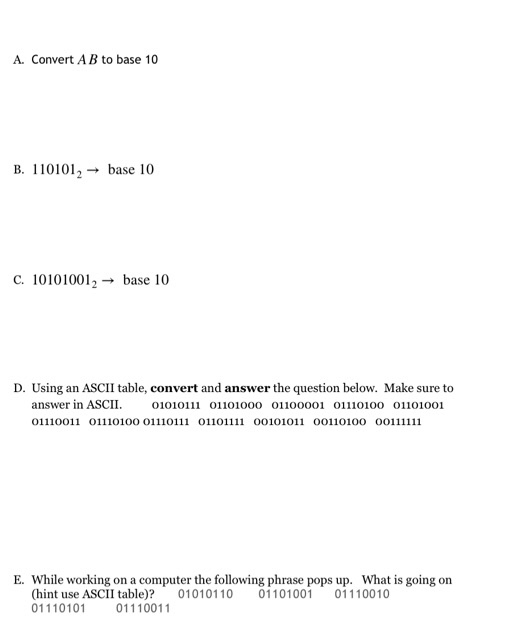A. Convert A B to base 10 B. 110101,→ base 10 C. 101010012base 10 D n am ASl tble cver and aner o answer in ASCII. 01010111 01101000 01100001 01110100 01101001 E. While working on a computer the following phrase pops up. What is going on (hint use ASCII table)? 01010110 01101001 01110010 01110101 01110011 Show transcribed image text A. Convert A B to base 10 B. 110101,→ base 10 C. 101010012base 10 D n am ASl tble cver and aner o answer in ASCII. 01010111 01101000 01100001 01110100 01101001 E. While working on a computer the following phrase pops up. What is going on (hint use ASCII table)? 01010110 01101001 01110010 01110101 01110011

Answer to A. Convert A B to base 10 B. 110101,→ base 10 C. 101010012base 10 D n am ASl tble cver and aner o answer in ASCII. 010…

## (Solved) : Altitude Ft Sea Level Corresponding Time Sec Fictitious Rocket Measured Follows Time 0 20 Q37188640 . . .

Write C code to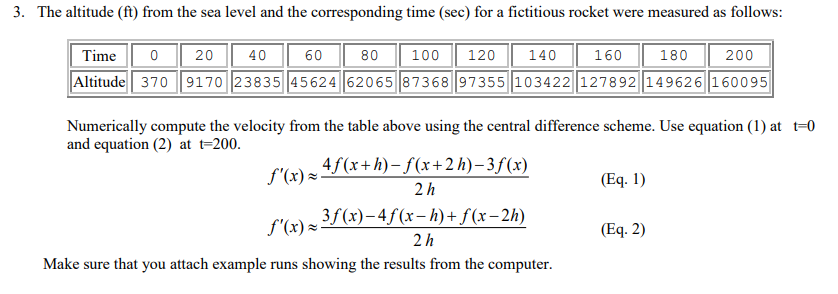. The altitude (ft) from the sea level and the corresponding time (sec) for a fictitious rocket were measured as follows: Time 0 20 40 6080100120140160180200 Altitude370 9170 23835 45624 62065 87368 97355 103422 127892 149626160095 Numerically compute the velocity from the table above using the central difference scheme. Use equation (1) at t-0 and equation (2) at t 200 f(x) Eq. 1 2 h f(x) Eq. 2) 2 h Make sure that you attach example runs showing the results from the computer Show transcribed image text . The altitude (ft) from the sea level and the corresponding time (sec) for a fictitious rocket were measured as follows: Time 0 20 40 6080100120140160180200 Altitude370 9170 23835 45624 62065 87368 97355 103422 127892 149626160095 Numerically compute the velocity from the table above using the central difference scheme. Use equation (1) at t-0 and equation (2) at t 200 f(x) Eq. 1 2 h f(x) Eq. 2) 2 h Make sure that you attach example runs showing the results from the computer

Answer to . The altitude (ft) from the sea level and the corresponding time (sec) for a fictitious rocket were measured as follows…

## (Solved) : Computer Uses Memory Unit 256k Words 32 Bits Binary Instruction Code Stored One Word Mem Q37197086 . . .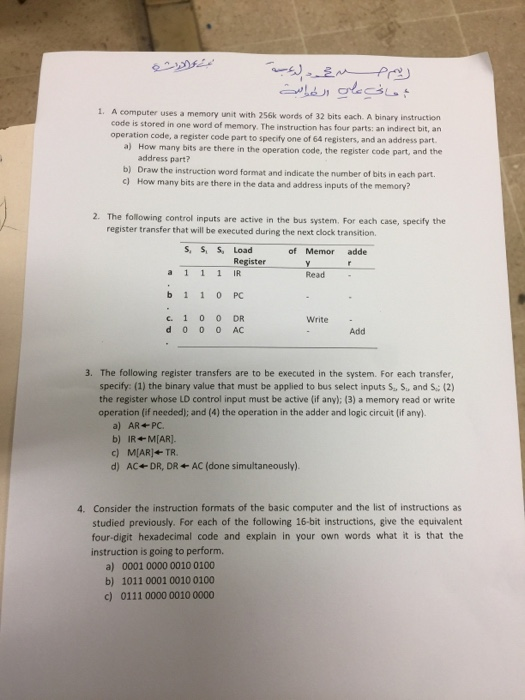. A computer uses a memory unit with 256k words of 32 bits each. A binary instruction code is stored in one word of memory. The instruction has four parts: an indirect bit, an operation code, a register code part to specify one of 64 registers, and an address part. a) How many bits are there in the operation code, the register code part, and the address part? b) Draw the instruction word format and indicate the number of bits in each pant c) How many bits are there in the data and address inputs of the memory? 2. The following control inputs are active in the bus system. For each case, speify the S, SS Load of Memor adde register transfer that will be executed during the next clock transition Register a 1 1 1 IR b11o PC c. 1 0 0 DR Read Write 000АС Add 3. The following register transfers are to be executed in the system. For each transfer, specify: (1) the binary value that must be applied to bus select inputs S, S, and S;(2) the register whose LD control input must be active (if anyl: (3) a memory read or write operation (if needed); and (4) the operation in the adder and logic circuit (if any) a) AR PC b) IR+ MIAR] c) M[AR]←TR. d) AC DR, DR AC (done simultaneously). 4. Consider the instruction formats of the basic computer and the list of instructions as studied previously. For each of the following 16-bit instructions, give the equivalent four-digit hexadecimal code and explain in your own words what it is that the instruction is going to perform. a) 0001 0000 0010 0100 b) 1011 0001 0010 0100 c) 0111 0000 0010 0000 Show transcribed image text . A computer uses a memory unit with 256k words of 32 bits each. A binary instruction code is stored in one word of memory. The instruction has four parts: an indirect bit, an operation code, a register code part to specify one of 64 registers, and an address part. a) How many bits are there in the operation code, the register code part, and the address part? b) Draw the instruction word format and indicate the number of bits in each pant c) How many bits are there in the data and address inputs of the memory? 2. The following control inputs are active in the bus system. For each case, speify the S, SS Load of Memor adde register transfer that will be executed during the next clock transition Register a 1 1 1 IR b11o PC c. 1 0 0 DR Read Write 000АС Add 3. The following register transfers are to be executed in the system. For each transfer, specify: (1) the binary value that must be applied to bus select inputs S, S, and S;(2) the register whose LD control input must be active (if anyl: (3) a memory read or write operation (if needed); and (4) the operation in the adder and logic circuit (if any) a) AR PC b) IR+ MIAR] c) M[AR]←TR. d) AC DR, DR AC (done simultaneously). 4. Consider the instruction formats of the basic computer and the list of instructions as studied previously. For each of the following 16-bit instructions, give the equivalent four-digit hexadecimal code and explain in your own words what it is that the instruction is going to perform. a) 0001 0000 0010 0100 b) 1011 0001 0010 0100 c) 0111 0000 0010 0000

Answer to . A computer uses a memory unit with 256k words of 32 bits each. A binary instruction code is stored in one word of memo…

## (Solved) : Build Linear Regression Model Gre Score Chance Admit Training Data Plot Regression Line Q37216966 . . .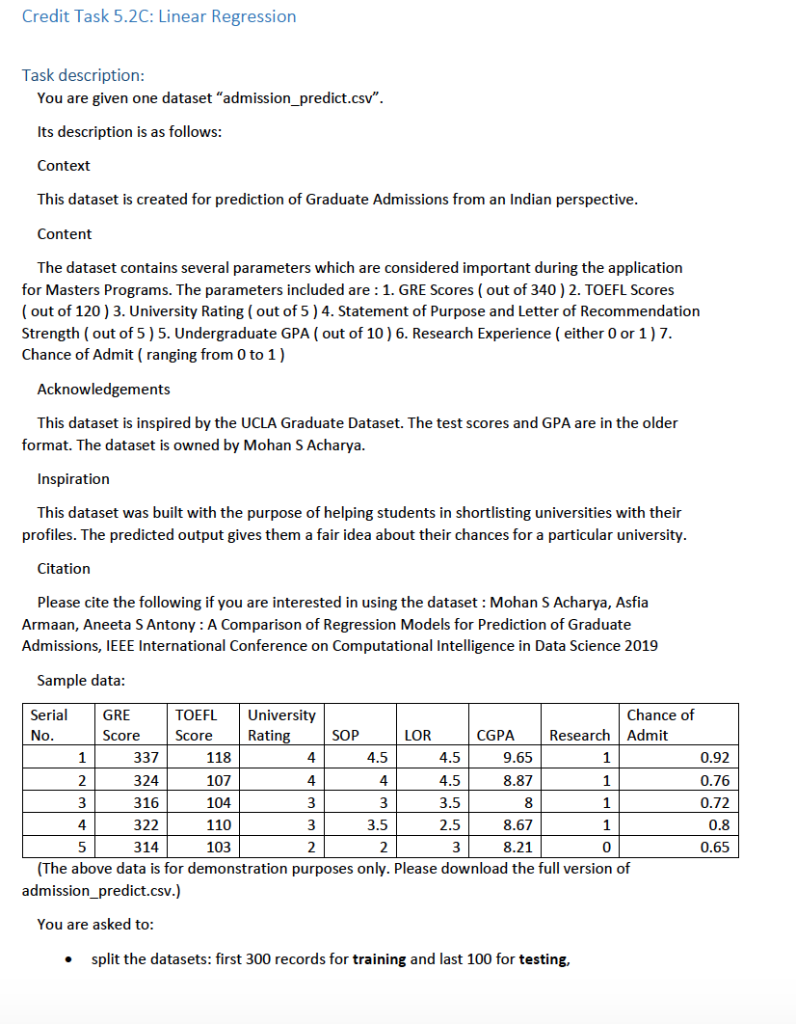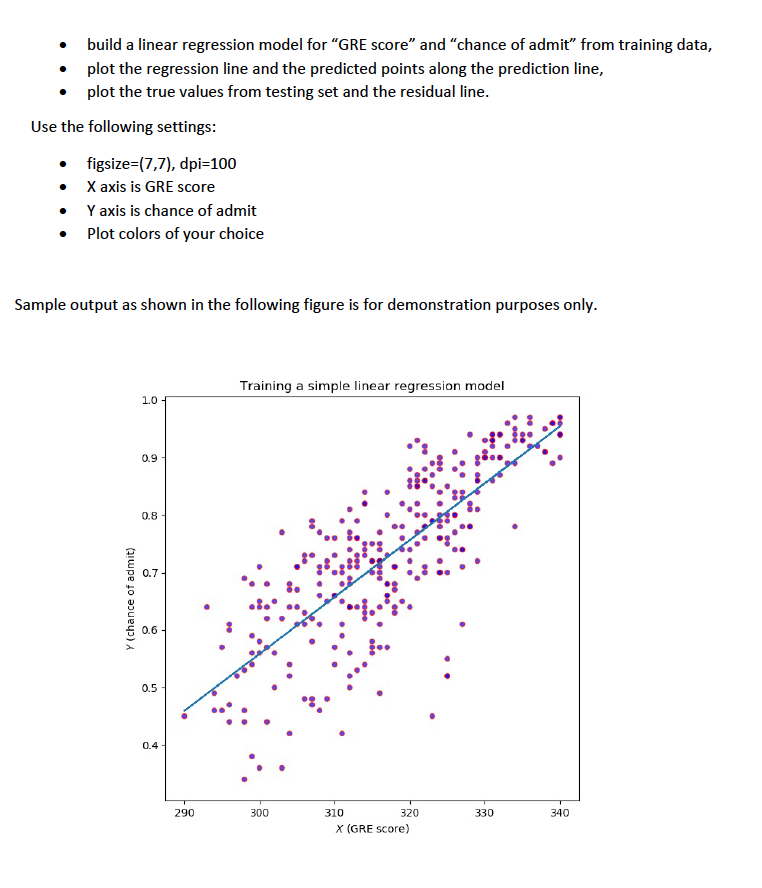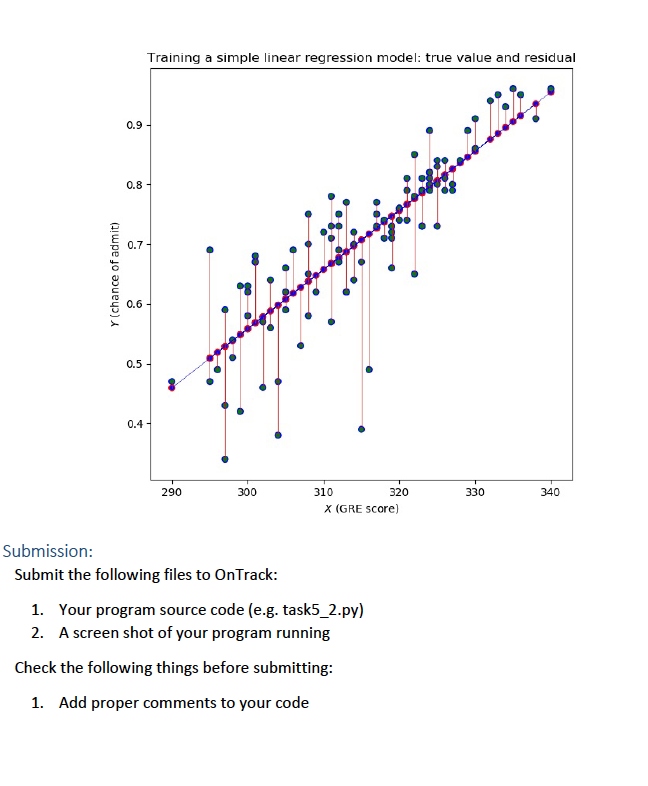We were unable to transcribe this image. build a linear regression model for “GRE score” and “chance of admit” from training data, . plot the regression line and the predicted points along the prediction line, . plot the true values from testing set and the residual line Use the following settings . figsize-(7,7), dpi-100 X axis is GRE score Y axis is chance of admit . Plot colors of your choice Sample output as shown in the following figure is for demonstration purposes only Training a simple linear regression model 1.0 0.9 ใน 0.8 D 0.7 0.5 0.4 300 320 290 310 330 340 x (GRE score) Training a simple linear regression model: true value and residual 0.9 0.8 0.7 E 0.6 0.5 0.4 290 300 310 320 330 340 X (GRE Score) Submission: Submit the following files to OnTrack: Your program source code (e.g. task5_2.py) A screen shot of your program running 1. 2. Check the following things before submitting Add proper comments to your code 1. Show transcribed image text
. build a linear regression model for “GRE score” and “chance of admit” from training data, . plot the regression line and the predicted points along the prediction line, . plot the true values from testing set and the residual line Use the following settings . figsize-(7,7), dpi-100 X axis is GRE score Y axis is chance of admit . Plot colors of your choice Sample output as shown in the following figure is for demonstration purposes only Training a simple linear regression model 1.0 0.9 ใน 0.8 D 0.7 0.5 0.4 300 320 290 310 330 340 x (GRE score)
Training a simple linear regression model: true value and residual 0.9 0.8 0.7 E 0.6 0.5 0.4 290 300 310 320 330 340 X (GRE Score) Submission: Submit the following files to OnTrack: Your program source code (e.g. task5_2.py) A screen shot of your program running 1. 2. Check the following things before submitting Add proper comments to your code 1.

Answer to . build a linear regression model for “GRE score” and “chance of admit” from training data, . plot the regression line a…

## (Solved) : Consider Surface Defined Z 4×2 Y2 Draw Traces Slices K Planes Y B Sketch Surface K Plane Q37296096 . . .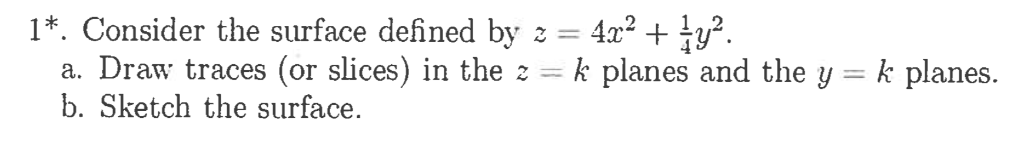* . Consider the surface defined by z-4×2 + Ỉy2. a. Draw traces (or slices) in the k planes and the y b. Sketch the surface. k planes. Show transcribed image text * . Consider the surface defined by z-4×2 + Ỉy2. a. Draw traces (or slices) in the k planes and the y b. Sketch the surface. k planes.

Answer to * . Consider the surface defined by z-4×2 + Ỉy2. a. Draw traces (or slices) in the k planes and the y b. Sketch the su…

## (Solved) : Create Class Call Collegecourse Add Field Department Course Number Section Number Maximum Q37227063 . . .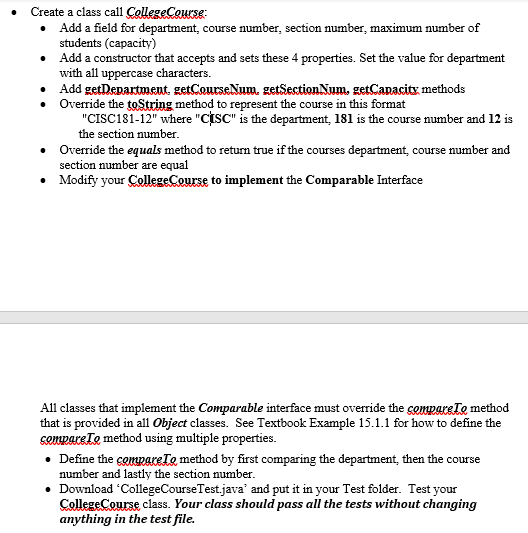Answer to . Create a class call CollegeCourse Add a field for department, course number, section number, maximum number of student…

## (Solved) : Apply Prim S Algorithm Graph Figure Priority Queue Include Vertices Already Tree Draw Min Q37288086 . . .

A. Apply Prim’s algorithm to the graph in the figure. In thepriority queue, include all the vertices that are not already inthe tree. Then draw the minimum spanning tree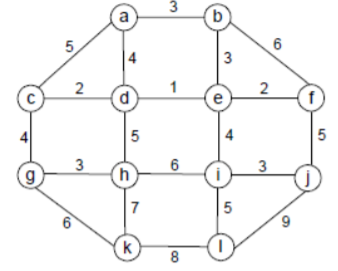B. Starting at vertex A, apply Dijkstra’s algorithm to the graphin the same figure above. In the priority queue, inlcude all thevertices that are not already in the tree. Also, list all shortestpaths and their lengths from the source vertex

5 9 6 2 3 3 5 8 3 4 s~ Show transcribed image text 5 9 6 2 3 3 5 8 3 4 s~

Answer to A. Apply Prim’s algorithm to the graph in the figure. In the priority queue, include all the vertices that are not alr…

## (Solved) : Convert B Base 10 B 110101 Base 10 C 101010012base 10 D N Asl Tble Cver Aner O Answer Asc Q37240061 . . .A. Convert A B to base 10 B. 110101,→ base 10 C. 101010012base 10 D n am ASl tble cver and aner o answer in ASCII. 01010111 01101000 01100001 01110100 01101001 E. While working on a computer the following phrase pops up. What is going on (hint use ASCII table)? 01010110 01101001 01110010 01110101 01110011 Show transcribed image text A. Convert A B to base 10 B. 110101,→ base 10 C. 101010012base 10 D n am ASl tble cver and aner o answer in ASCII. 01010111 01101000 01100001 01110100 01101001 E. While working on a computer the following phrase pops up. What is going on (hint use ASCII table)? 01010110 01101001 01110010 01110101 01110011

Answer to A. Convert A B to base 10 B. 110101,→ base 10 C. 101010012base 10 D n am ASl tble cver and aner o answer in ASCII. 010…

## (Solved) : Consider Following Javascript Code Function Maker B C Var X B Var Y B C Return Function M Q37232491 . . .

​​​​​​​

. Consider thefollowing Javascript code,

functionmaker(a,b,c) {

var x = a + b;

var y = b * c;

return function(m,n) {       var k =x – m;       return k * n;

}

}

var cl = maker (3,2,1);

var f =cl(4,5);

What is thereferencing environment inside cl(4,5)? What are the value of thevariables in the referencing environment?

Answer to ​​​​​​​ . Consider the following Javascript code, function maker(a,b,c) { var x = a + b; var y = b * c; re…

## App

We are the best freelance writing portal. Looking for online writing, editing or proofreading jobs? We have plenty of writing assignments to handle.